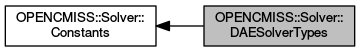OpenCMISS-Iron FORTRAN API Documentation
OPENCMISS::Solver::DAESolverTypes

The differential-algebraic equation solver types for a differential-algebraic equation solver. More...

Collaboration diagram for OPENCMISS::Solver::DAESolverTypes:## Variables

integer(intg), parameter, public opencmiss_iron::cmfe_solver_dae_euler = SOLVER_DAE_EULER
Euler differential-algebraic equation solver. More...

integer(intg), parameter, public opencmiss_iron::cmfe_solver_dae_crank_nicolson = SOLVER_DAE_CRANK_NICOLSON
Crank-Nicolson differential-algebraic equation solver. More...

integer(intg), parameter, public opencmiss_iron::cmfe_solver_dae_runge_kutta = SOLVER_DAE_RUNGE_KUTTA
Runge-Kutta differential-algebraic equation solver. More...

integer(intg), parameter, public opencmiss_iron::cmfe_solver_dae_adams_moulton = SOLVER_DAE_ADAMS_MOULTON
Adams-Moulton differential-algebraic equation solver. More...

integer(intg), parameter, public opencmiss_iron::cmfe_solver_dae_bdf = SOLVER_DAE_BDF
General BDF differential-algebraic equation solver. More...

integer(intg), parameter, public opencmiss_iron::cmfe_solver_dae_rush_larson = SOLVER_DAE_RUSH_LARSON
Rush-Larson differential-algebraic equation solver. More...

integer(intg), parameter, public opencmiss_iron::cmfe_solver_dae_external = SOLVER_DAE_EXTERNAL
External (e.g., CellML generated) differential-algebraic equation solver. More...

## Detailed Description

The differential-algebraic equation solver types for a differential-algebraic equation solver.

See also
OPENCMISS::Solver::Constants,OPENCMISS

## Variable Documentation

 integer(intg), parameter, public opencmiss_iron::cmfe_solver_dae_adams_moulton = SOLVER_DAE_ADAMS_MOULTON

Adams-Moulton differential-algebraic equation solver.

See also

Definition at line 6218 of file opencmiss_iron.f90.

 integer(intg), parameter, public opencmiss_iron::cmfe_solver_dae_bdf = SOLVER_DAE_BDF

General BDF differential-algebraic equation solver.

See also

Definition at line 6219 of file opencmiss_iron.f90.

 integer(intg), parameter, public opencmiss_iron::cmfe_solver_dae_crank_nicolson = SOLVER_DAE_CRANK_NICOLSON

Crank-Nicolson differential-algebraic equation solver.

See also

Definition at line 6216 of file opencmiss_iron.f90.

 integer(intg), parameter, public opencmiss_iron::cmfe_solver_dae_euler = SOLVER_DAE_EULER

Euler differential-algebraic equation solver.

See also

Definition at line 6215 of file opencmiss_iron.f90.

 integer(intg), parameter, public opencmiss_iron::cmfe_solver_dae_external = SOLVER_DAE_EXTERNAL

External (e.g., CellML generated) differential-algebraic equation solver.

See also

Definition at line 6221 of file opencmiss_iron.f90.

 integer(intg), parameter, public opencmiss_iron::cmfe_solver_dae_runge_kutta = SOLVER_DAE_RUNGE_KUTTA

Runge-Kutta differential-algebraic equation solver.

See also

Definition at line 6217 of file opencmiss_iron.f90.

 integer(intg), parameter, public opencmiss_iron::cmfe_solver_dae_rush_larson = SOLVER_DAE_RUSH_LARSON

Rush-Larson differential-algebraic equation solver.

See also

Definition at line 6220 of file opencmiss_iron.f90.